# Derivation Bisics

28 Jan 2021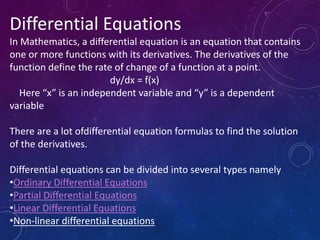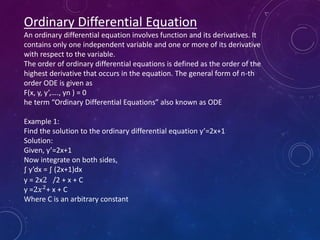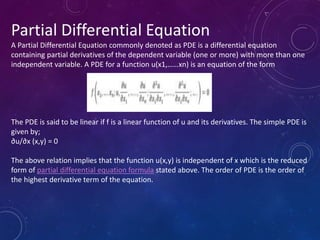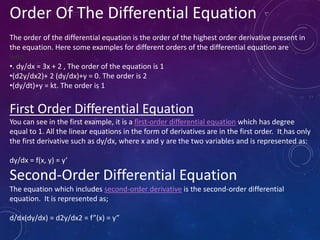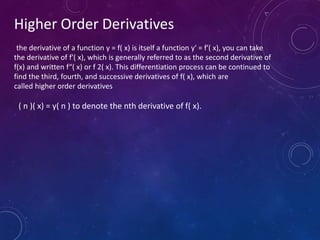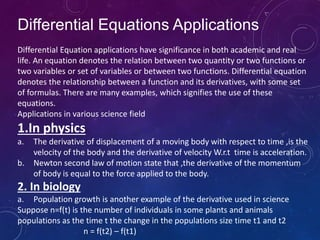1 sur 10

### Derivation Bisics

• 1. Differential Equations In Mathematics, a differential equation is an equation that contains one or more functions with its derivatives. The derivatives of the function define the rate of change of a function at a point. dy/dx = f(x) Here “x” is an independent variable and “y” is a dependent variable There are a lot ofdifferential equation formulas to find the solution of the derivatives. Differential equations can be divided into several types namely •Ordinary Differential Equations •Partial Differential Equations •Linear Differential Equations •Non-linear differential equations
• 2. Ordinary Differential Equation An ordinary differential equation involves function and its derivatives. It contains only one independent variable and one or more of its derivative with respect to the variable. The order of ordinary differential equations is defined as the order of the highest derivative that occurs in the equation. The general form of n-th order ODE is given as F(x, y, y’,…., yn ) = 0 he term “Ordinary Differential Equations” also known as ODE Example 1: Find the solution to the ordinary differential equation y’=2x+1 Solution: Given, y’=2x+1 Now integrate on both sides, ∫ y’dx = ∫ (2x+1)dx y = 2x2 /2 + x + C y =2𝑥2 + x + C Where C is an arbitrary constant
• 3. Partial Differential Equation A Partial Differential Equation commonly denoted as PDE is a differential equation containing partial derivatives of the dependent variable (one or more) with more than one independent variable. A PDE for a function u(x1,……xn) is an equation of the form The PDE is said to be linear if f is a linear function of u and its derivatives. The simple PDE is given by; ∂u/∂x (x,y) = 0 The above relation implies that the function u(x,y) is independent of x which is the reduced form of partial differential equation formula stated above. The order of PDE is the order of the highest derivative term of the equation.
• 4. How to Represent Partial Differential Equation? In PDEs, we denote the partial derivatives using subscripts, such as; In some cases, like in Physics when we learn about wave equations or sound equation, partial derivative, ∂ is also represented by ∇(del or nabla).
• 5. Order Of The Differential Equation The order of the differential equation is the order of the highest order derivative present in the equation. Here some examples for different orders of the differential equation are g iven. •. dy/dx = 3x + 2 , The order of the equation is 1 •(d2y/dx2)+ 2 (dy/dx)+y = 0. The order is 2 •(dy/dt)+y = kt. The order is 1 First Order Differential Equation You can see in the first example, it is a first-order differential equation which has degree equal to 1. All the linear equations in the form of derivatives are in the first order. It has only the first derivative such as dy/dx, where x and y are the two variables and is represented as: dy/dx = f(x, y) = y’ Second-Order Differential Equation The equation which includes second-order derivative is the second-order differential equation. It is represented as; d/dx(dy/dx) = d2y/dx2 = f”(x) = y”
• 6. Higher Order Derivatives the derivative of a function y = f( x) is itself a function y′ = f′( x), you can take the derivative of f′( x), which is generally referred to as the second derivative of f(x) and written f“( x) or f 2( x). This differentiation process can be continued to find the third, fourth, and successive derivatives of f( x), which are called higher order derivatives f( n )( x) = y( n ) to denote the nth derivative of f( x).
• 7. Differential Equations Applications Differential Equation applications have significance in both academic and real life. An equation denotes the relation between two quantity or two functions or two variables or set of variables or between two functions. Differential equation denotes the relationship between a function and its derivatives, with some set of formulas. There are many examples, which signifies the use of these equations. Applications in various science field 1.In physics a. The derivative of displacement of a moving body with respect to time ,is the velocity of the body and the derivative of velocity W.r.t time is acceleration. b. Newton second law of motion state that ,the derivative of the momentum of body is equal to the force applied to the body. 2. In biology a. Population growth is another example of the derivative used in science Suppose n=f(t) is the number of individuals in some plants and animals populations as the time t the change in the populations size time t1 and t2 n = f(t2) – f(t1)
• 8. In chemistry A .One use of derivatives in chemistry is when you want to find out the concentrations Of an element in a priducts b. Derivative is used to find the rate of reaction and compressability in chemistry And many more uses in chemistry that are beyond the approach at basic level In mathematics Is used to find; Extreme value of functions The means value theorms Monotonic functions Curves sketching Newton and methods etc.
• 9. In other sciences a) Rate of heat flow in geology. b) Rate of spread of roumors in sociology c) Rate of improvement of performance in psychology d) Inshort ,, Derivatives is widely used in every field of life and in every field of science almost , to find how much the rate of change of particular process or function.
• 10. THANK YOU